# Confusion Matrix in Machine Learning

In the field of machine learning and specifically the problem of statistical classification, a confusion matrix, also known as an error matrix. A confusion matrix is a table that is often used to describe the performance of a classification model (or “classifier”) on a set of test data for which the true values are known. It allows the visualization of the performance of an algorithm.
It allows easy identification of confusion between classes e.g. one class is commonly mislabeled as the other. Most performance measures are computed from the confusion matrix.

1. What the confusion matrix is and why you need to use it.
2. How to calculate a confusion matrix for a 2-class classification problem from scratch.
3. How to create a confusion matrix in Python.

Confusion Matrix:
A confusion matrix is a summary of prediction results on a classification problem. The number of correct and incorrect predictions are summarized with count values and broken down by each class. This is the key to the confusion matrix. The confusion matrix shows the ways in which your classification model is confused when it makes predictions. It gives us insight not only into the errors being made by a classifier but more importantly the types of errors that are being made.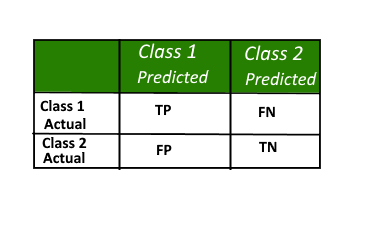Here,

• Class 1 : Positive
• Class 2 : Negative

Definition of the Terms:

• Positive (P) : Observation is positive (for example: is an apple).
• Negative (N) : Observation is not positive (for example: is not an apple).
• True Positive (TP) : Observation is positive, and is predicted to be positive.
• False Negative (FN) : Observation is positive, but is predicted negative.
• True Negative (TN) : Observation is negative, and is predicted to be negative.
• False Positive (FP) : Observation is negative, but is predicted positive.

Classification Rate/Accuracy:
Classification Rate or Accuracy is given by the relation: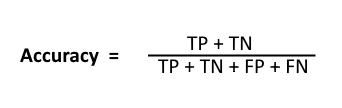However, there are problems with accuracy. It assumes equal costs for both kinds of errors. A 99% accuracy can be excellent, good, mediocre, poor or terrible depending upon the problem.

Recall: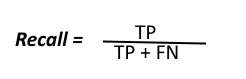Recall can be defined as the ratio of the total number of correctly classified positive examples divide to the total number of positive examples. High Recall indicates the class is correctly recognized (a small number of FN).

Precision: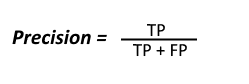To get the value of precision we divide the total number of correctly classified positive examples by the total number of predicted positive examples. High Precision indicates an example labelled as positive is indeed positive (a small number of FP).

High recall, low precision: This means that most of the positive examples are correctly recognized (low FN) but there are a lot of false positives.

Low recall, high precision: This shows that we miss a lot of positive examples (high FN) but those we predict as positive are indeed positive (low FP)

F-measure:
Since we have two measures (Precision and Recall) it helps to have a measurement that represents both of them. We calculate an F-measure which uses Harmonic Mean in place of Arithmetic Mean as it punishes the extreme values more.
The F-Measure will always be nearer to the smaller value of Precision or Recall.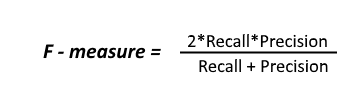Let’s consider an example now, in which we have infinite data elements of class B and a single element of class A and the model is predicting class A against all the instances in the test data.
Here,

`Precision : 0.0`
`Recall : 1.0`

Now:
`Arithmetic mean: 0.5`
`Harmonic mean: 0.0`
When taking the arithmetic mean, it would have 50% correct. Despite being the worst possible outcome! While taking the harmonic mean, the F-measure is 0.

Example to interpret confusion matrix: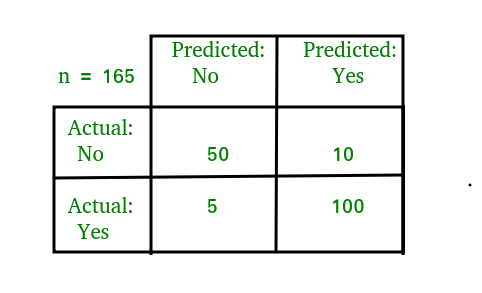For the simplification of the above confusion matrix I have added all the terms like TP, FP, etc and the row and column totals in the following image: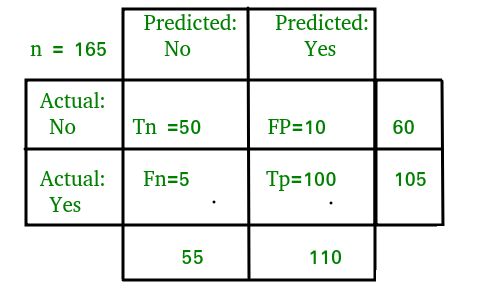Now,
Classification Rate/Accuracy:
`Accuracy = (TP + TN) / (TP + TN + FP + FN) = (100 + 50) /(100 + 5 + 10 + 50) = 0.90`

Recall: Recall gives us an idea about when it’s actually yes, how often does it predict yes.
`Recall = TP / (TP + FN) = 100 / (100 + 5) = 0.95`

Precision: Precsion tells us about when it predicts yes, how often is it correct.
`Precision = TP / (TP + FP)=100/ (100+10) = 0.91`

F-measure:
`Fmeasure = (2 * Recall * Precision) / (Recall + Presision) = (2 * 0.95 * 0.91) / (0.91 + 0.95) = 0.92`

Here is a python script which demonstrates how to create a confusion matrix on a predicted model. For this, we have to import the confusion matrix module from sklearn library which helps us to generate the confusion matrix.

Code : Python code to explain the above explanation

 `# Python script for confusion matrix creation. ` `from` `sklearn.metrics ``import` `confusion_matrix ` `from` `sklearn.metrics ``import` `accuracy_score ` `from` `sklearn.metrics ``import` `classification_report ` ` `  `actual ``=` `[``1``, ``1``, ``0``, ``1``, ``0``, ``0``, ``1``, ``0``, ``0``, ``0``] ` `predicted ``=` `[``1``, ``0``, ``0``, ``1``, ``0``, ``0``, ``1``, ``1``, ``1``, ``0``] ` `results ``=` `confusion_matrix(actual, predicted) ` ` `  `print` `'Confusion Matrix :'` `print``(results) ` `print` `'Accuracy Score :'``,accuracy_score(actual, predicted) ` `print` `'Report : '` `print` `classification_report(actual, predicted) `

Output:

```Confusion Matrix :
[[4 2]
[1 3]]
Accuracy Score : 0.7
Report :
precision    recall  f1-score   support
0       0.80      0.67      0.73         6
1       0.60      0.75      0.67         4
avg / total       0.72      0.70      0.70        10
```

Note that this program might not run on Geeksforgeeks IDE, but it can run easily on your local python interpreter, provided, you have installed the required libraries.

This article is contributed by Abhishek Sharma. If you like GeeksforGeeks and would like to contribute, you can also write an article using contribute.geeksforgeeks.org or mail your article to contribute@geeksforgeeks.org. See your article appearing on the GeeksforGeeks main page and help other Geeks.